This page provides the online reproducibility of the figures in the paper:

### Revisiting the directional mutation pressure theory: the analysis of a particular genomic structure in Leishmania major

#### A. Necşulea and J.R. Lobry

Abstract:
This paper reports the existence of a significant negative correlation between GC12 and GC3 in the recently sequenced genome of Leishmania major. This result contradicts the previous evidence that the compositional correlations between codon positions are universal. Moreover, it challenges the interpretation of the GC12 vs GC3 linear regression slope as the relative neutrality of GC12, within the framework of the directional mutation pressure theory (Sueoka, 1988).
The analysis of the codon usage pattern for L. major shows that codon choice is most likely influenced by both mutation pressure and translational selection. Dinucleotide frequencies were also analysed; our results do not support the existence of an unusual neighbour-dependent mutation bias in this genome.
We developed two evolutionary models that could explain the origin of the negative GC12/GC3 correlation. The first model is based on the effect of translational selection on the GC3; the second one is based on a potential mutation bias combined with purifying selection at the amino-acid level. Both models predict a negative GC12/GC3 correlation at the equilibrium.
The potential implications of these results for this aspect of the directional mutation pressure theory are discussed. We conclude that the particular case of L. major should lead to a careful reevaluation of several hypotheses of this theory. The origin of the negative GC12/GC3 correlation remains for now an open question.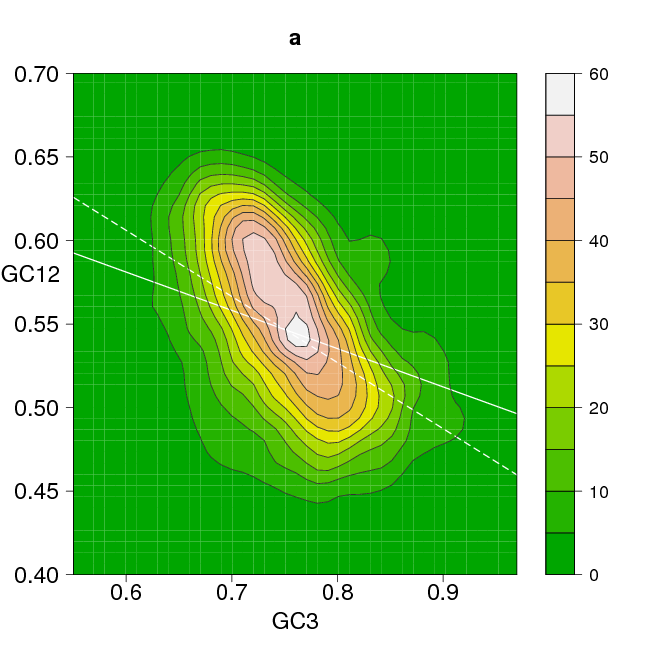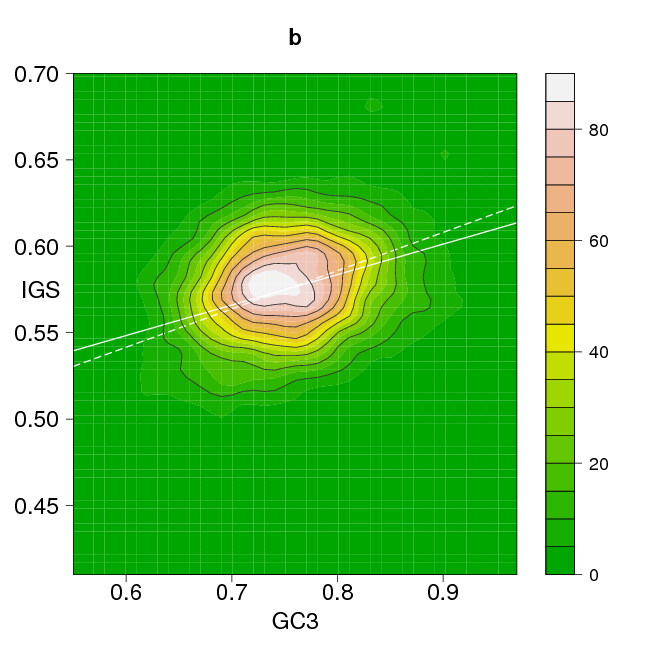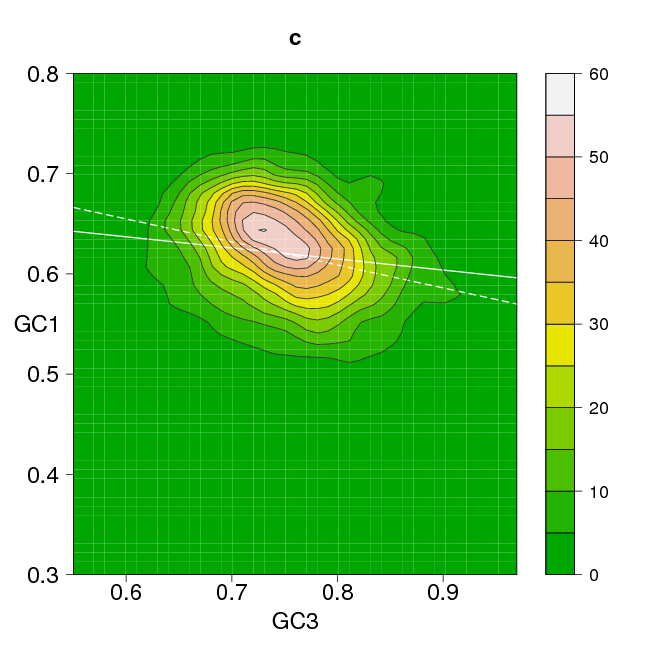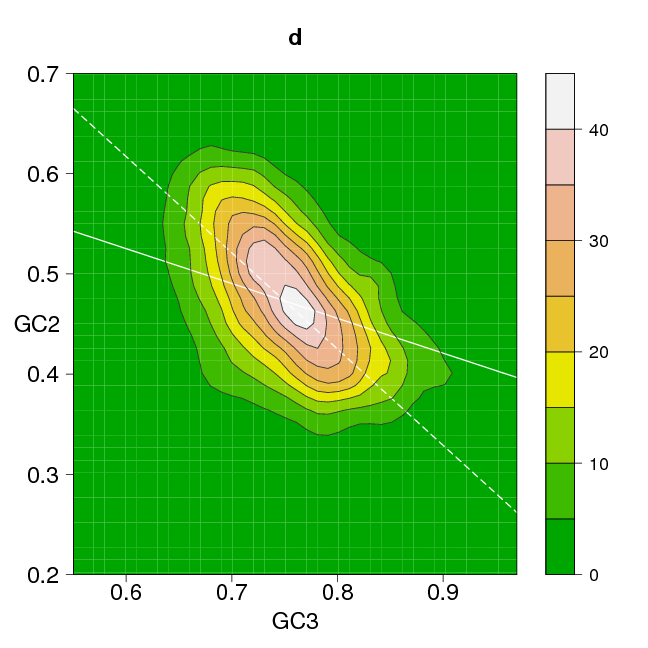Figure 1. Sueoka's neutrality plots for Leishmania major. The solid line corresponds to the linear regression (against GC3) and the dashed line corresponds to the orthogonal regression between the two variables. a) GC12 vs GC3, Spearman correlation rho = -0.4; b) Intergenic GC vs. GC3, rho = 0.26; c) GC1 vs. GC3, rho = -0.22; d) GC2 vs. GC3, rho = -0.42.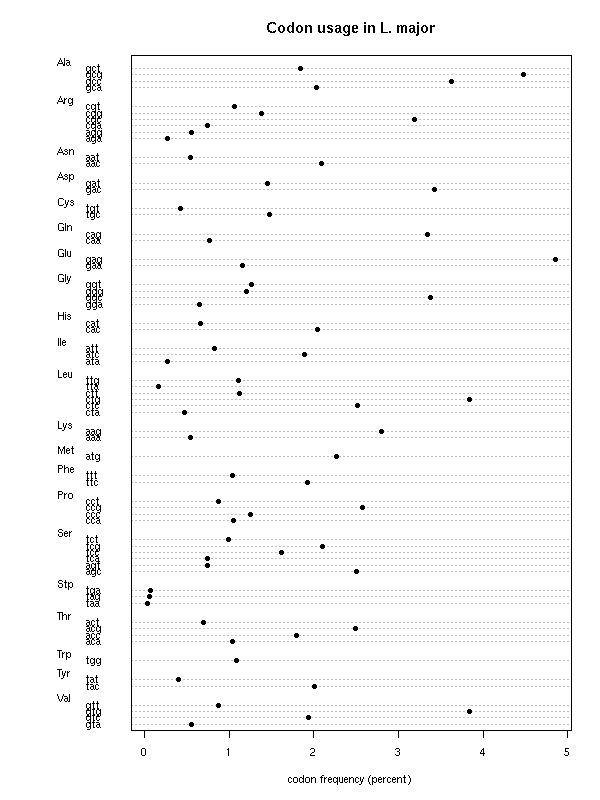Figure 2. Codon frequencies (percentages) for 8260 L. major protein-coding genes.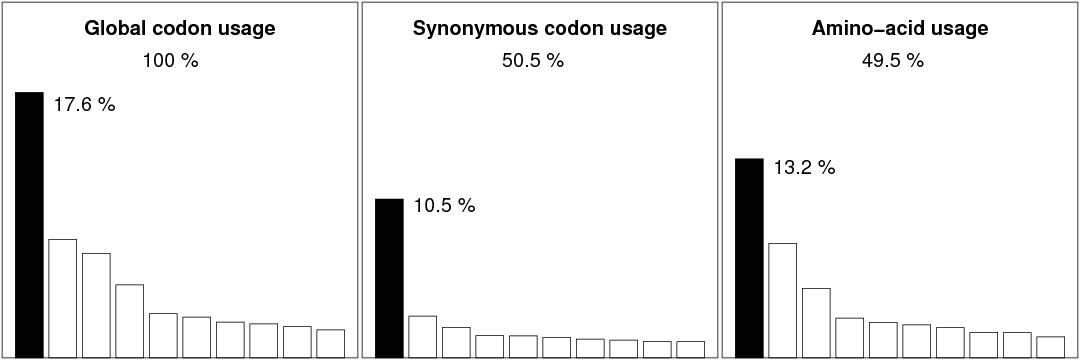Figure 3. Decomposition of the total variability of codon usage into synonymous and non-synonymous effects. Only the contributions of the first 10 factors of each COA are represented graphically. Effects at the synonymous level are responsible for 50.5 % of the total variability, the remaining 49.5 % correspond to effects at the non-synonymous level. The first factor for the global codon usage COA, the synonymous codon usage COA and the non-synonymous COA explain respectively 17.6 %, 10.5 % and 13.2 % of the total variability.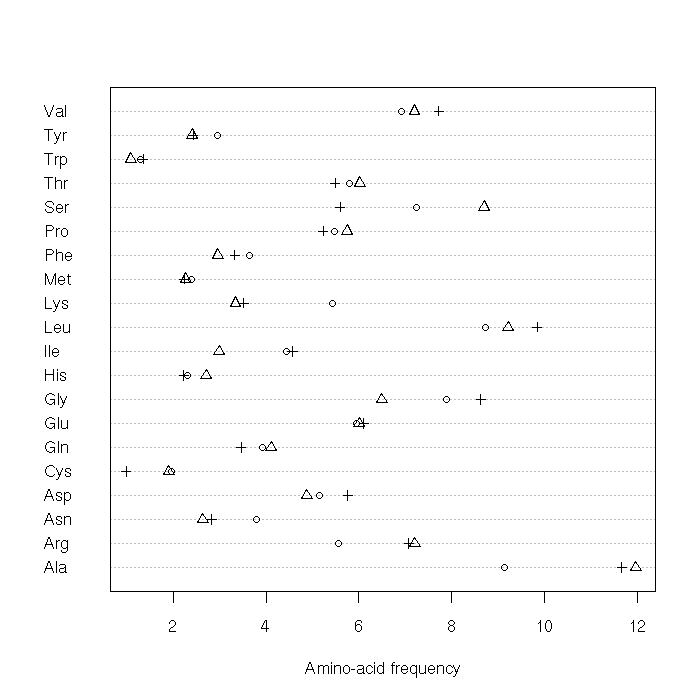Figure 4. Comparison between the pooled amino-acid usage of L. major proteins and the pooled amino-acid usage from prokaryotic and eukaryotic genes with a GC3 content higher than 65 %, from D'Onofrio et al., 1999. Values on the X axis represent the amino-acid frequency (in %); triangles correspond to frequencies in L. major, crosses represent prokaryotes and circles represent eukaryotes.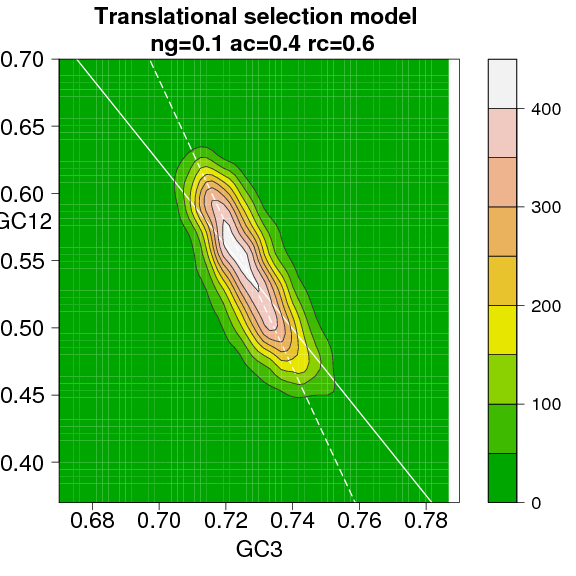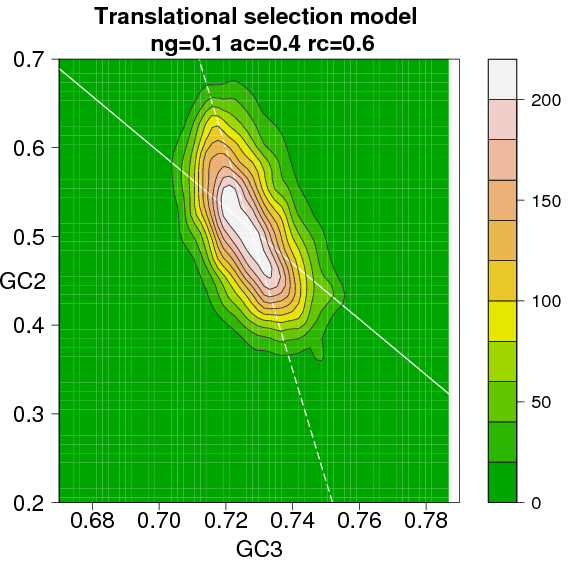Figure 5. Translational selection model: prediction of the equilibrium state, when ng = 0.1, ac = 0.4 and rc = 0.6. The solid line corresponds to the linear regression and the dashed line corresponds to the orthogonal regression between the two variables. Left: GC12 vs. GC3 plot, Spearman correlation is rho = -0.75. Right: GC2 vs. GC3 plot, Spearman correlation is rho = -0.51.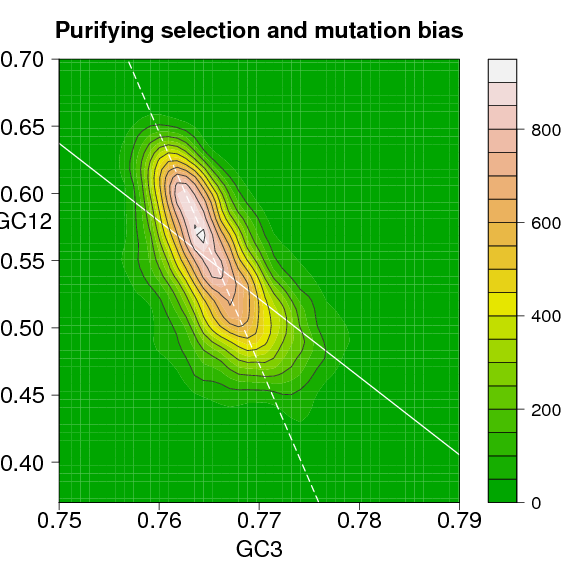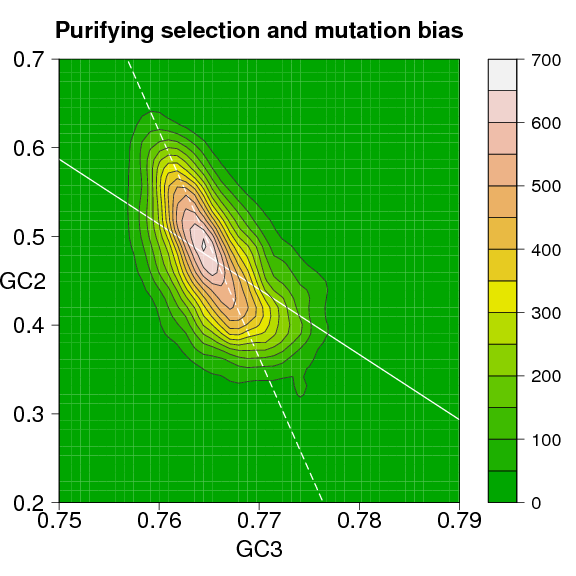Figure 6. Purifying selection and mutation bias model: prediction of the equilibrium state, when s = 0, alpha1 = 0.025, beta1 = 0.01, gamma1 = 3.5 and gamma2 = 1.5. The solid line corresponds to the linear regression and the dashed line corresponds to the orthogonal regression between the two variables. Left: GC12 vs. GC3 plot, Spearman correlation is rho = -0.62. Right: GC2 vs. GC3 plot, Spearman correlation is rho = -0.58.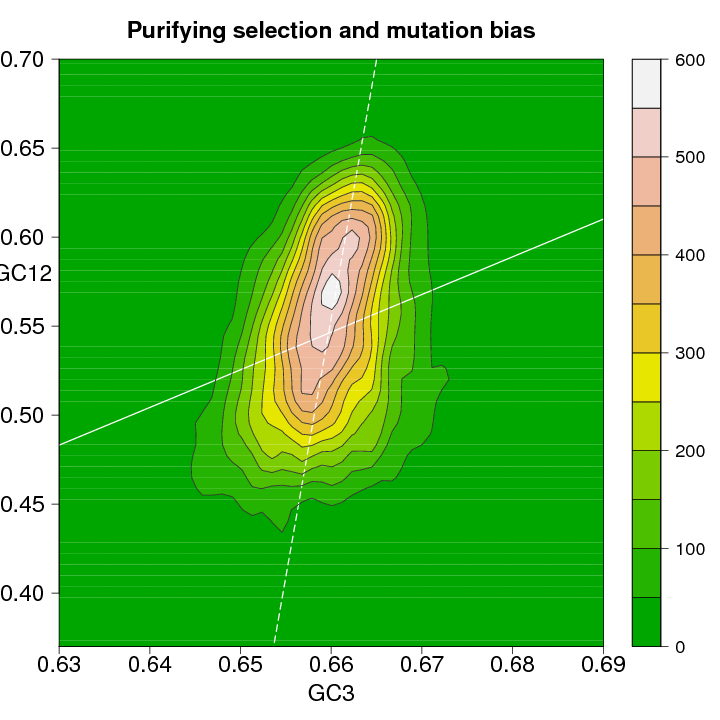Figure 7. Purifying selection and mutation bias model: prediction of the equilibrium state, when s = 0, alpha1 = 0.025, beta1 = 0.01, gamma1 = 1.5 and gamma2 = 3.5. The solid line corresponds to the linear regression and the dashed line corresponds to the orthogonal regression between the two variables. The Spearman correlation between GC12 and GC3 is rho = 0.3.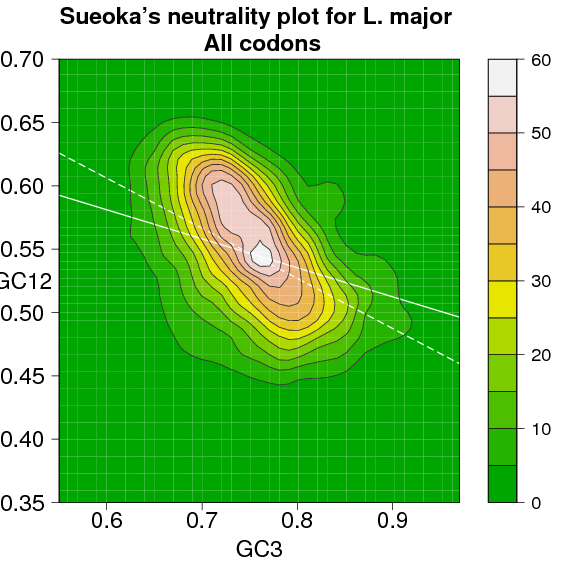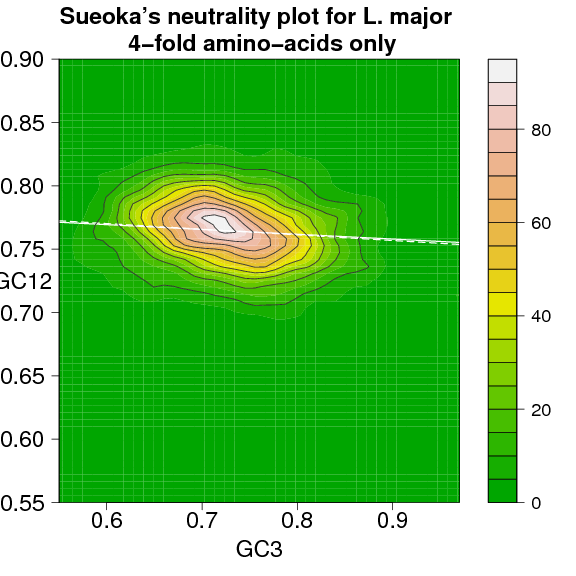Figure 8. Comparison between Sueoka's neutrality plots for L. major, when all codons are considered (left) and when only 4-fold amino-acids are considered (right). In the first case the correlation between GC12 and GC3 is rho = -0.4, while in the second case rho = -0.15. The axes scale are the same for both plots. The solid line corresponds to the linear regression and the dashed line corresponds to the orthogonal regression between the two variables.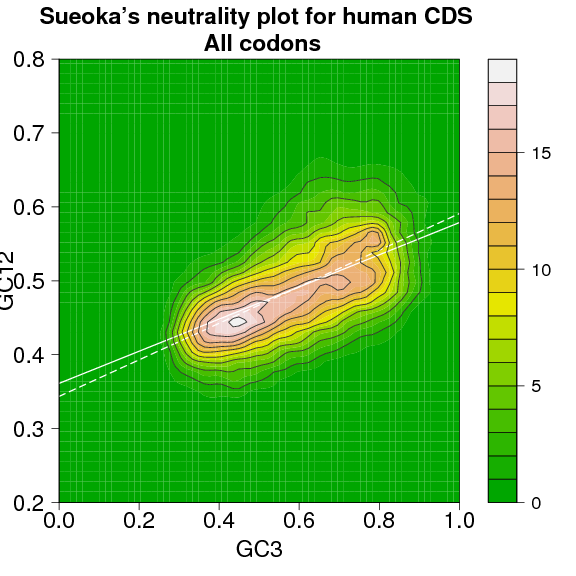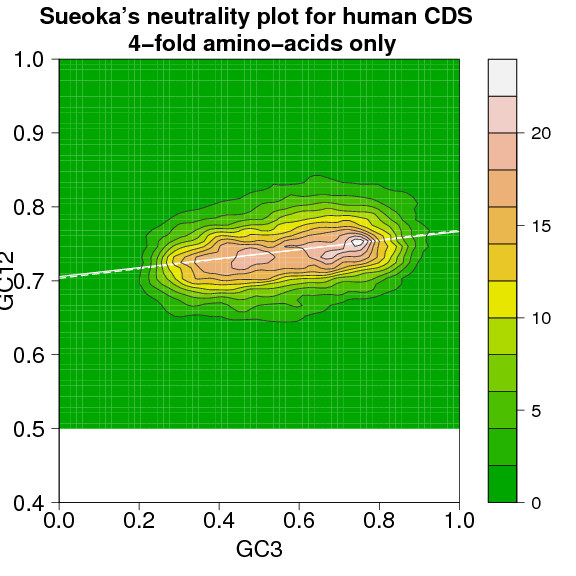Figure 9. Comparison between Sueoka's neutrality plots for human CDS, when all codons are considered (left) and when only 4-fold amino-acids are considered (right). In the first case the correlation between GC12 and GC3 is rho = 0.56 while in the second case rho = 0.26. The axes scale are the same for both plots.